Courses

# Test: Bond Dissociation Enthalpy

## 18 Questions MCQ Test Chemistry for JEE | Test: Bond Dissociation Enthalpy

Description
This mock test of Test: Bond Dissociation Enthalpy for JEE helps you for every JEE entrance exam. This contains 18 Multiple Choice Questions for JEE Test: Bond Dissociation Enthalpy (mcq) to study with solutions a complete question bank. The solved questions answers in this Test: Bond Dissociation Enthalpy quiz give you a good mix of easy questions and tough questions. JEE students definitely take this Test: Bond Dissociation Enthalpy exercise for a better result in the exam. You can find other Test: Bond Dissociation Enthalpy extra questions, long questions & short questions for JEE on EduRev as well by searching above.
QUESTION: 1

Solution:
QUESTION: 2

### ΔfH° of CS2 = 117.36 kJ mol-1 C(g) = 716.682 kJ mol-1 S(g) = 278.805 kJ mol-1  Q. Thus,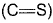bond enthalpy in CS2 is

Solution:

∆H = (B.E)reactant - (B.E)product
117.36 = 716.682 + 2(278.805) - ∆H2C=S
∆H 2C=S = 1156.932
∆HC=S = 1156.932/2 = 578.74 kJ mol-1

QUESTION: 3

### The dissociation energy of CH4 and C2H6 to convert them into gaseous atoms are 360 and 620 kcal mol-1 respectively. Thus, bond energy of (C—C) bond is

Solution:

In CH4 there are 4-C-H bonds.
Let C-H bond energy be X. So 4X = 360. or X = 90.
Now, in C2H6 there are 6 C-H bonds and 1C-C bond.
Let C-C B. E be y.
So 6X + Y = 620. or Y = 620-6×90 = 80.

QUESTION: 4

The (C— Cl) bond energy of CCI4 (l) can be derived from thermochemical data

Solution:
QUESTION: 5

Bond dissociation energy of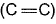= + 590 kJ mol-1 and that of (C—C) = + 331 kJ mol-1 at 298 K.

Enthalpy change for the polymerisation of ethene to polyethene is (where n is large integral value)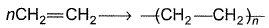Solution:

The correct answer is option B
During the polymerization of ethylene, one mole of ethylene breaks i.e. one C = C double bond breaks and the two CH2- groups are linked with C-C single bonds thus forming three single bonds.

But in the whole unit of polymer, number of single C-C bonds formed/mole of ethylene is 2.

Energy due to formation of 2 C-C single bonds = 2 X 331 = 662 kJ/mol.

Energy due to dissociation of 1  C = C double bond = 590 kJ/mol.

Thus, the enthalpy of polymerisation per mole of ethylene at 298 K = (590-662) = -72 kJ/mol

QUESTION: 6

Given at 298 K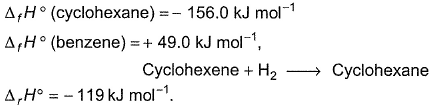Q. The resonance energy of benzene is

Solution:

C6H10(l) + H2(g) → C6H12(l) , ∆hydrogenationH° = -119 kJ mol-1
6C(s) + 6H2(g) → C6H12(l) , ∆fH° = -156 kJ mol-1
6C(s) + 3H2(g)  → C6H6(l) , ∆fH° = +49 kJ mol-1
C6H6(l) → C6H12(l) , ∆hydrogenationH°
(∆hydrogenationH°)Actual = -156-49 = -2055 kJ mol-1
Hypothetical heat of        = (heat of hydrogenation = 3 × (Heat of hydrogenation
hydrogenation of benzene   of cyclohexatriene) of cyclohexene)
= 3(-119) = -357 kJmol-1
Here, we have assumed that there are only double bonds in benzene(Kekule structure) and in hydrogenating it, energy released will be roughly 3 times of hydrogenating cyclohexene
Clearly, heat of hydrogenation, for theoretical benzene is more negative and thus it is less stable. So, resonance energy should be negative(since actual benzene is stable)
Resonance energy = -357 -(-205) = -152 kJ mol-1

QUESTION: 7

Given the following thermochemical data at 298 K and 1 bar

ΔH°vap (CH3OH) = 38.0 kJ mol-1
ΔfH°: H(g) = 218 kJ mol-1
O(g) = 249 kJ mol-1
C(g) = 715 kJ mol-1

Bond dissociation energy

(C—H) = 415 kJ mol-1
(C—O) = 356 kJ mol-1
(O—H) = 4 6 3 kJ mol-1

Q. The ΔfH° of liquid methyl alcohol in kJ mol-1 is

Solution:
QUESTION: 8

Heat of hydrogenation of ethene is x1 and that of benzene is x2. The resonance energy of benzene is

Solution:

Energy of ethene =x1
Number of ethene in benzene=3.
Therefore, Observed energy of hydrogenation of benzene =3x1
Calculated energy of hydrogenation of benzene =x2 (Given)
Therefore,
Resonance energy = observed - calculated =3x1−x2

QUESTION: 9

Given, BE of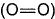= 498.8 kJ mol-1
BE of (O—O) in ozone = 302.3 kJ mol-1

Q. What is enthalpy change of the reaction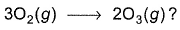Solution:

3O2(g) → 2O3(g)
∆H = 3(B.E)O2 - 2(B.E)O3
= 3(498.8) - 2(302.3)
= 287.2 kJ ml-1

QUESTION: 10

The standard enthalpies of formation of SF6 (g), S (g)and F (g) are -1100, + 275 and + 80 kJ mol-1. Thus, average bond energy of (S—F) in SF6 is

Solution:

S(g) + 6F(g) → SF6(g)
∆rH = ∑∆Hreactant -  ∑∆Hproduct
= (275+6 80) - (-1100)
= 1855
So,  bond energy of (S—F) = 1855/6 = 309.16 kJ mol-1

QUESTION: 11

Calculate resonance energy of N2O from the following data

ΔfH° (N2O) = 82 kJ mol-1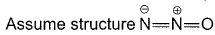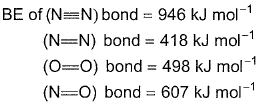Solution:

= 946 + 249 - 607 - 418 = 1195 - 1025 = 170 kJ mol-1
Resonance energy = Observed heat of formation – calculated heat of formation
= 82 -170 = 88 kJ mol-1

QUESTION: 12

Given, ΔfH° (H2S) = 20.1 kJ mol-1

ΔfH° (H)(g) = +218.0 kJ mol-1
ΔfH° (S) (g) = +277.0 kJ mol-1

and bond dissociation energy of first (H—S) bond in H2S is 376.6 kJ mol-1. Thus, ΔfH° (HS)is

Solution:
QUESTION: 13

Given, BE (H—H) - x1, BE= x2, BE (O—H) = x3 and for H2O (l) → H2O (g), ΔH = x4 mol-1, then ΔfH° [H2O (l)] is

Solution:

Required reaction= Heat of formation of water – H2(g) +1/2O2 (g)= H2O(l)
WRITE THE EQUATION PROPERLY FOR EACH EQUATION
rearranging the reactions we have to get the above equation
B.E. of H--H= H2(g) → 2H(g)..........X1
B.E of O2 = O2(g) ---> 2O(g)...........X2
B.E. OF O-H = O-H(aq)--> O(g)+H(g) …......X3
LATENT HEAT OF VAPOURISATION OF WATER = H2O(l)----> H2O(g)..........X4
THUS REVERSING EQTN X3 AND X4 , HALVING EQUATION X2 AND DOUBLING EQUATION X3 WE WILL GET THE DESIRED EQUATION.

QUESTION: 14

Direction (Q. Nos. 14 -15) This section contains a paragraph, wach describing  theory, experiments, data etc. three Questions related to paragraph have been  given.Each question have only one correct answer among the four given  ptions  (a),(b),(c),(d).

Based on the following thermochemical data of the given process, answer the questions.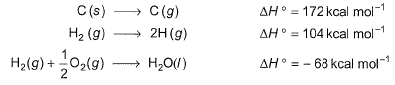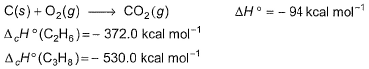Q. Bond energy of (C— C) bond is

Solution:

For C3H8 : 3C + 4H2 → C3H8 ; ∆H1
For C3H8 : 2C + 3H2 → C2H6 ; ∆H2
∆H1 = -[2(C-C)+8(C-H)]+[3Cs→g+4(H-H)]     -(I)
∆H2 = -[1(C-C)+6(C-H)]+[2Cs→g+3(H-H)]     -(2)
Let bond energy of C-C be x kcal and bond energy of C-H be y  kcal.
From eqn I and 2
∆H1 = -[2x+8y]+[3×172+4×104]     -(3)
∆H2 = -[x+6y]+[2×172+3×104]     -(4)
Also given, C+O2 → CO2; ∆H = -94.0 kcal     -(5)
H2 + ½ O2 → H2O ; ∆H = -68.0 kcal      -(6)
C2H6 + 7/2 O2 → 2CO2 + 3H2O ; ∆H = -372 kcal     -(7)
C3H8 + 5O2  → 3CO2 + 4H2O ; ∆H = -530 kcal-(8)
By inspection method,2 × (5) + 3 × (6) - (7) gives
2C + 3H2 → C2H6 ; ∆H2 = -20 kcal
And  3×(5) + 4×(6) -(8)
3C + 4H2 → C3H8 ; ∆H3 = -20 kcal
By eqn. (3), (4), (9) and (10)
x+6y = 676
2x+8y = 956
x = 82 kcal and y = 99 kcal

QUESTION: 15

Based on the following thermochemical data of the given process, answer the questions.Q. Bond energy of (C—H) bond is

Solution:

For C3H8 : 3C + 4H2 → C3H8 ; ∆H1
For C3H8 : 2C + 3H2 → C2H6 ; ∆H2
∆H1 = -[2(C-C)+8(C-H)]+[3Cs→g+4(H-H)]     -(I)
∆H2 = -[1(C-C)+6(C-H)]+[2Cs→g+3(H-H)]     -(2)
Let bond energy of C-C be x kcal and bond energy of C-H be y  kcal.
From eqn I and 2
∆H1 = -[2x+8y]+[3×172+4×104]     -(3)
∆H2 = -[x+6y]+[2×172+3×104]     -(4)
Also given, C+O2 → CO2; ∆H = -94.0 kcal     -(5)
H2 + ½ O2 → H2O ; ∆H = -68.0 kcal      -(6)
C2H6 + 7/2 O2 → 2CO2 + 3H2O ; ∆H = -372 kcal     -(7)
C3H8 + 5O2  → 3CO2 + 4H2O ; ∆H = -530 kcal     -(8)
By inspection method,  2 × (5) + 3 × (6) - (7) gives
2C + 3H2 → C2H6 ; ∆H2 = -20 kcal
And  3×(5) + 4×(6) -(8)
3C + 4H2 → C3H8 ; ∆H3 = -20 kcal
By eqn. (3), (4), (9) and (10)
x+6y = 676
2x+8y = 956
x = 82 kcal and y = 99 kcal

*Answer can only contain numeric values
QUESTION: 16

Direction (Q. Nos. 16 and 17) This section contains 2 questions. when worked out will result in an integer from 0 to 9 (both inclusive).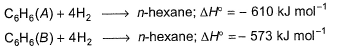Given bond dissociation energy under standard states.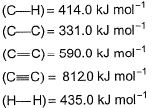Q. i. Number of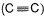andbonds in (A) is ......

Solution:

Let us assume the structures as given below and calculate bond energies,
Let us assume the given structures of C6H6 are: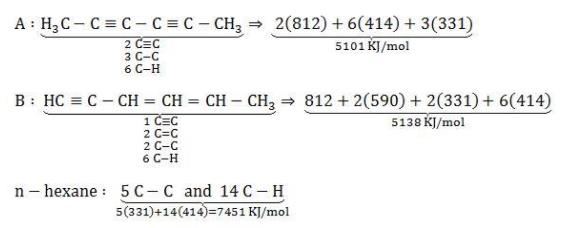A + 4H2  ---> n - hexane
H =5101+4(435) -7451 = -610KJ/mol
B+4H2 ---> n - hexane
H =5138+4(435)-74551 =-573KJ/mol
Hence our assumed structures are correct.

*Answer can only contain numeric values
QUESTION: 17Given bond dissociation energy under standard states.Q. ii. Number of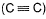and) bond in (B) is .......

Solution:

Number of bonds between C and C are 3
So the correct answer is 3.

QUESTION: 18

Direction (Q. Nos. 18) Choice the correct combination of elements and column I and coloumn II  are given as option (a), (b), (c) and (d), out of which ONE option is correct.

Q. Given thermochemical data under standard states at 278 K and 1 bar.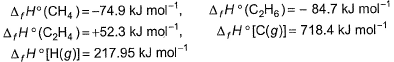Match the types of bonds in Column I with their respective value of bond-energy in Column II and select answer from the codes given below the table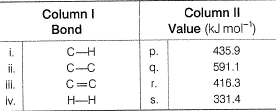Solution:

∆H = ∑(B.E)reactant - ∑(B.E)product
For CH4
C(g) + 4H(g) → CH4
∆H = (718.4) + 4(217.95) - (-74.9)
4 C-H  = 1665.1
C-H = 416.3
For C2H6
2C(g) + 6H(g) → C2H6(g)
∆H = 2(718.4) + 6(217.95) - (-84.7)
C-C+ 6C-H = 2828.5
C-C = (2828.5 - 2497.8) = 331.4
From here, we can see that option c is correct.